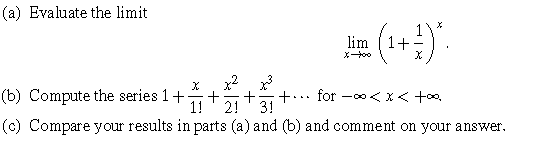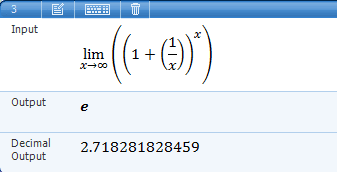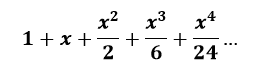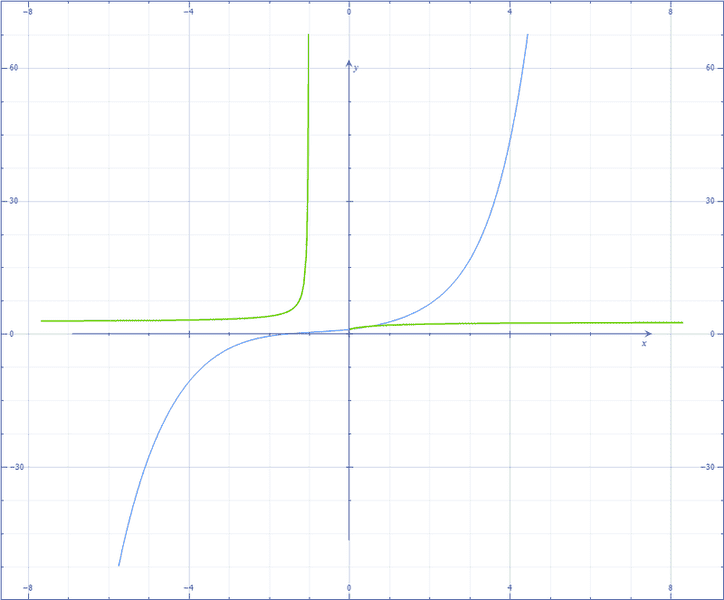# Software assignment - Evaluate the limit & compare the series

A.Q
Software assignment -- Evaluate the limit & compare the series

## Homework Statement## The Attempt at a Solution

In part a, I used Microsoft mathematics and found it eIn part b, I used $\sum$ but I can't find the right formula
I don't want an easy answer but I realy have no idea about part b, it's not even in the textbook

## Answers and Replies

Staff Emeritus
Try computing the first few terms of the series. Or write a little spreadsheet that will do it for you.

A.Q
Try computing the first few terms of the series. Or write a little spreadsheet that will do it for you.

Is it right?Staff Emeritus
Obviously you cannot evaluate the series for every point between -∞ and ∞, but you can plot it for a subset of that range.

A.Q
Obviously you cannot evaluate the series for every point between -∞ and ∞, but you can plot it for a subset of that range.

I can't plot it as a function because the power of x increases till infinity.
Could you explain me how to plot that series, please?

dirk_mec1
Indeed you can NOT plot all terms so you need to cut it somewhere to get a feeling to which value it is going.

Staff Emeritus
You labeled this as a software assignment, so presumably you are supposed to write some kind of software to evaluate the series.

A.Q
Indeed you can NOT plot all terms so you need to cut it somewhere to get a feeling to which value it is going.

to which term should I cut it, I think every term makes a great difference

A.Q
You labeled this as a software assignment, so presumably you are supposed to write some kind of software to evaluate the series.

Right, I'm supposed to use Microsoft mathematics or Maxima to answer this question so how can I do it using a sophisticated calculator, what is the mathematic operation?

Staff Emeritus
to which term should I cut it, I think every term makes a great difference
Does it? Try it with x equal to a small number. You should see those terms quickly start dropping. Then try with a slightly larger number, say x=10. Now it's going to take a while, 15 or 20 terms, before they start dropping. But then they will drop quickly. Now try -10.

No matter how big you make x, those terms will eventually start dropping. Have you studied series in a mathematics class?

I would suggest keeping x to a smallish range. -20 to 20, for example, or even smaller. You will find that the value of the series grows large quite rapidly as x gets larger and larger.

Staff Emeritus
Right, I'm supposed to use Microsoft mathematics or Maxima to answer this question so how can I do it using a sophisticated calculator, what is the mathematic operation?
You have Mathematica? Just let it evaluate the infinite series for you. Mathematica knows about Infinity.

jackmell

## Homework Statement## The Attempt at a Solution

In part a, I used Microsoft mathematics and found it eIn part b, I used $\sum$ but I can't find the right formula
I don't want an easy answer but I realy have no idea about part b, it's not even in the textbook

I'm assuming this is an exercise in using some type of CAS software such as Mathematica or other and not a programming exercise to compute these things numerically. So what you need is to come up with the summation formula for part b, that is:

$$\sum_{n=0}^{\infty} a_n x^n=1+x+\frac{x^2}{2}+\frac{x^3}{3!}+\cdots$$

well, $x^0=1$ and then they're all just increasing powers so part of it is just

$$\sum_{n=0}^{\infty}a_n x^n$$

and the others are just increasing factorial functions $\frac{1}{0!}, \frac{1}{1!}, \frac{1}{2!}$ and so on. So what's $a_n$ then?

Staff Emeritus
Homework Helper
The series in part b is one of the most recognizable there is. IDK what kind of book you are using, but I'm surprised it's not in there.

Anywho, you can evaluate the sum thru the first 10 terms or so with a calculator. I think you'll be amazed at the result.

Staff Emeritus
The series in part b is one of the most recognizable there is.
Mathematica and maple will recognize it right off the bat.

A.Q
I'm assuming this is an exercise in using some type of CAS software such as Mathematica or other and not a programming exercise to compute these things numerically. So what you need is to come up with the summation formula for part b, that is:

$$\sum_{n=0}^{\infty} a_n x^n=1+x+\frac{x^2}{2}+\frac{x^3}{3!}+\cdots$$

well, $x^0=1$ and then they're all just increasing powers so part of it is just

$$\sum_{n=0}^{\infty}a_n x^n$$

and the others are just increasing factorial functions $\frac{1}{0!}, \frac{1}{1!}, \frac{1}{2!}$ and so on. So what's $a_n$ then?

$a_n$=$1/n!$

The series in part b is one of the most recognizable there is. IDK what kind of book you are using, but I'm surprised it's not in there.

Anywho, you can evaluate the sum thru the first 10 terms or so with a calculator. I think you'll be amazed at the result.

I've plotted the first 10 terms and I was really amazed because there isn't any similarity between it and (1+$1/x$)$^{x}$how should I compare part a & b

Staff Emeritus
Homework Helper
What're you doing? This isn't a plotting exercise. You've got to find the SUM of the series in part b.

For part b, let x = 1 and sum up the first 10 terms or so. Compare that result with what you got in part a.
You can use Mathematica or MS Mathematics or the simple Calculator app. Takes less than 5 minutes.

Staff Emeritus
I didn't know you had Mathematica until post #9. (My post #10 was in response to a previous post.) Mathematica knows about factorials, and it knows about infinity (it's Infinity in Mathematica).

Just have it sum the series as expressed in the original post. Sum[x^n/n!, {n, 0, Infinity}] should do it.

•1 person
A.Q
I didn't know you had Mathematica until post #9. (My post #10 was in response to a previous post.) Mathematica knows about factorials, and it knows about infinity (it's Infinity in Mathematica).

Just have it sum the series as expressed in the original post. Sum[x^n/n!, {n, 0, Infinity}] should do it.

Thank you Mr. D H. that is exactly what I need

A.Q
What're you doing? This isn't a plotting exercise. You've got to find the SUM of the series in part b.

For part b, let x = 1 and sum up the first 10 terms or so. Compare that result with what you got in part a.
You can use Mathematica or MS Mathematics or the simple Calculator app. Takes less than 5 minutes.

I've got it but why x=1.
the question says -inf$\leq$x$\geq$inf . How I'm supposed to get the hint that when x=1 the series equals e